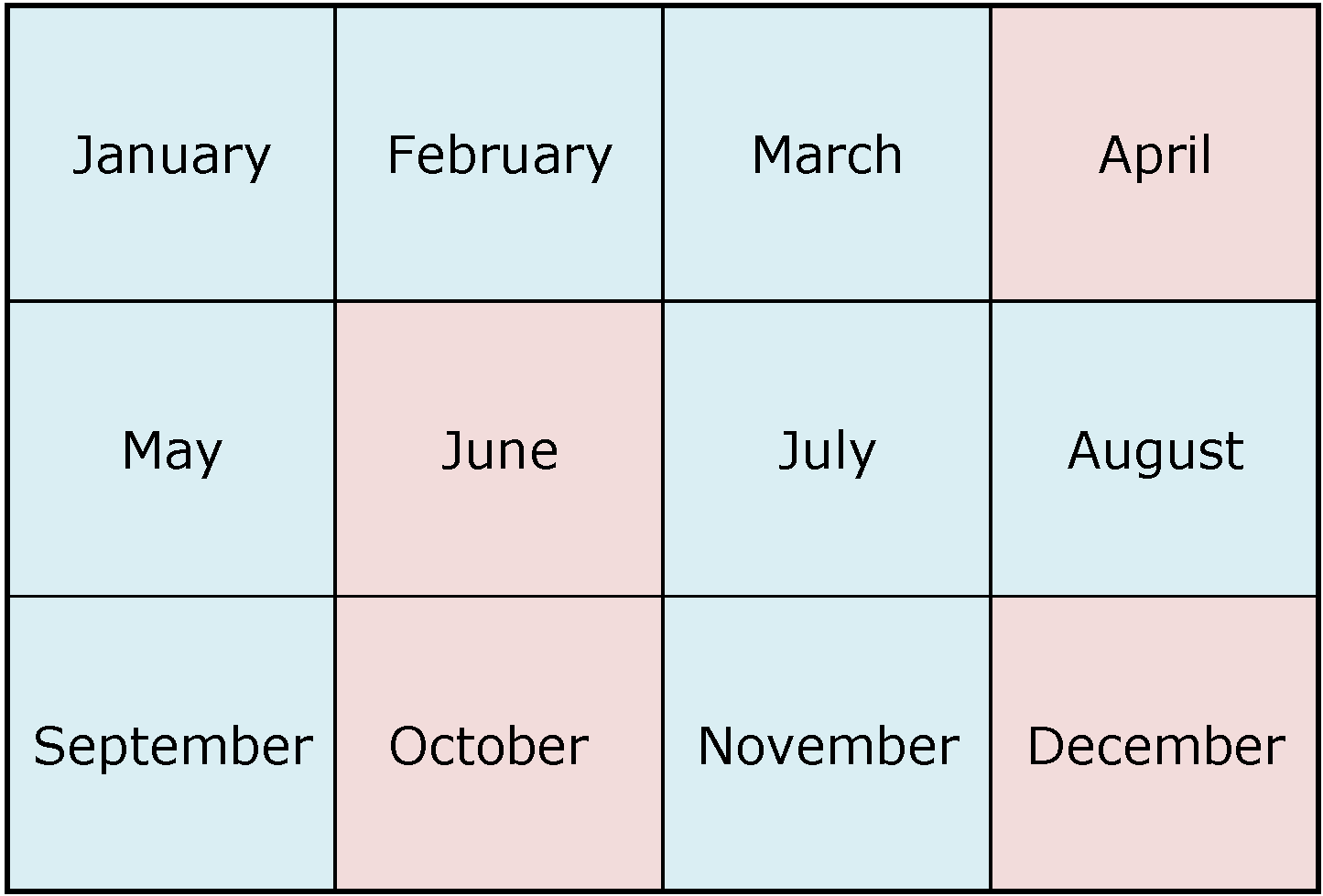ISSN 2284-7995, ISSN Online 2285-3952

## EVALUATION OF THE NITROGEN USE IN WHEAT CROP IN RELATION TO AMMONIUM NITRATE FERTILIZER

###### Published in Scientific Papers. Series "Management, Economic Engineering in Agriculture and rural development", Vol. 22 ISSUE 3 Written by Florin SALA

This study evaluated the use of nitrogen in wheat crop, in terms of Agronomic Efficiency (AE) and Partial Factor Productivity (PFP). The experiment was conducted in the specific conditions of the Banat University of Agricultural Science and Veterinary Medicine of Timisoara (BUASVM), Timis County, Romania. Nitrogen (N) was provided on the basis of ammonium nitrate, in the range 0 - 250 kg a.s. ha-1, in 11 experimental variants (T1 to T11). The AE index varied between 14.631 (T2) and 9.138 (T11), with a maximum (27.211) corresponding to the N75 dose (T4). The PFP index recorded decreasing values from 90.497 (T2) to 16.725 (T11). The AE index variation in relation to N was described by the polynomial equation of degree 4 (R2 = 0.975, p <0.01), and the AE index variation according to Y was described by a polynomial equation of degree 3 (R2 = 0.722, p = 0.0233). In relation to the calculated progressive increase yield (PY) the AE variation was described by a linear equation (R2 = 0.780, p <0.001). The PFP index variation in relation to N was described by a polynomial equation of degree 3 (R2 = 0.994, p <0.001), and in relation to N and Y, as simultaneous influence, was described in statistical safety conditions (R2 = 0.885, p = 0.0144).

SALA F. 2022, EVALUATION OF THE NITROGEN USE IN WHEAT CROP IN RELATION TO AMMONIUM NITRATE FERTILIZER. Scientific Papers. Series "Management, Economic Engineering in Agriculture and rural development", Vol. 22 ISSUE 3, PRINT ISSN 2284-7995, 609-616.### Find ArticleNext Issue will be published in AprilFour issues are published every year.

The publisher is not responsible for the opinions published in the Volume. They represent the authors’ point of view.

© 2019 To be cited: Scientific Papers. Series “Management, Economic Engineering in Agriculture and Rural Development“.